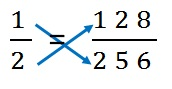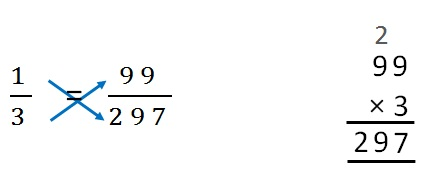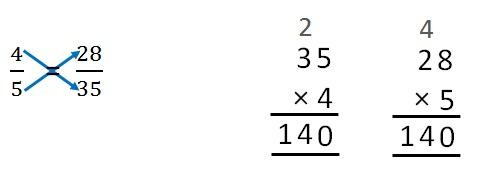Equivalent Fractions

Chapter 7 Class 6 Fractions
Concept wise

Let’s take an example

### Check whether 1/2 , 128/256 are equivalent .

To check,

we make both fractions equal.

1/2 = 128/256

And then cross multiply1 × 256 = 128 × 2

256 = 256

Since this is true

1/2, 128/256 are equivalent

### Check whether 1/ 3 , 99 /2 97 are equivalent.

To check,

we make both fractions equal.

1/3 = 99/297

And then cross multiply1 × 297 = 99 × 3

297 = 297

Since this is true

1/2, 99/297 are equivalent

### Check whether 4 / 5 , 28 / 35 are equivalent.

To check,

we make both fractions equal.

4/5 = 28/35

And then cross multiply4 × 35 = 28 × 5

140 = 140

Since this is true.

4/5, 28/35 are equivalent.

Learn in your speed, with individual attention - Teachoo Maths 1-on-1 Class

### Transcript

Checking whether fractions are equivalentLet’s take an example Check whether 𝟏/𝟐, 𝟏𝟐𝟖/𝟐𝟓𝟔 are equivalent. To check, we make both fractions equal. 1/2 = 128/256 And then cross multiply 1/2 = 128/256 1 × 256 = 128 × 2 256 = 256 Since this is true 1/2, 128/256 are equivalent Check whether 𝟏/𝟑, 𝟗𝟗/𝟐𝟗𝟕 are equivalent. To check, we make both fractions equal. 1/3 = 99/297 And then cross multiply 1/3 = 99/297 1 × 297 = 99 × 3 297 = 297 Since this is true 1/2, 99/297 are equivalent Check whether 𝟒/𝟓, 𝟐𝟖/𝟑𝟓 are equivalent. To check, we make both fractions equal. 4/5 = 28/35 And then cross multiply 4/5 = 28/35 4 × 35 = 28 × 5 140 = 140 Since this is true. 4/5, 28/35 are equivalent.